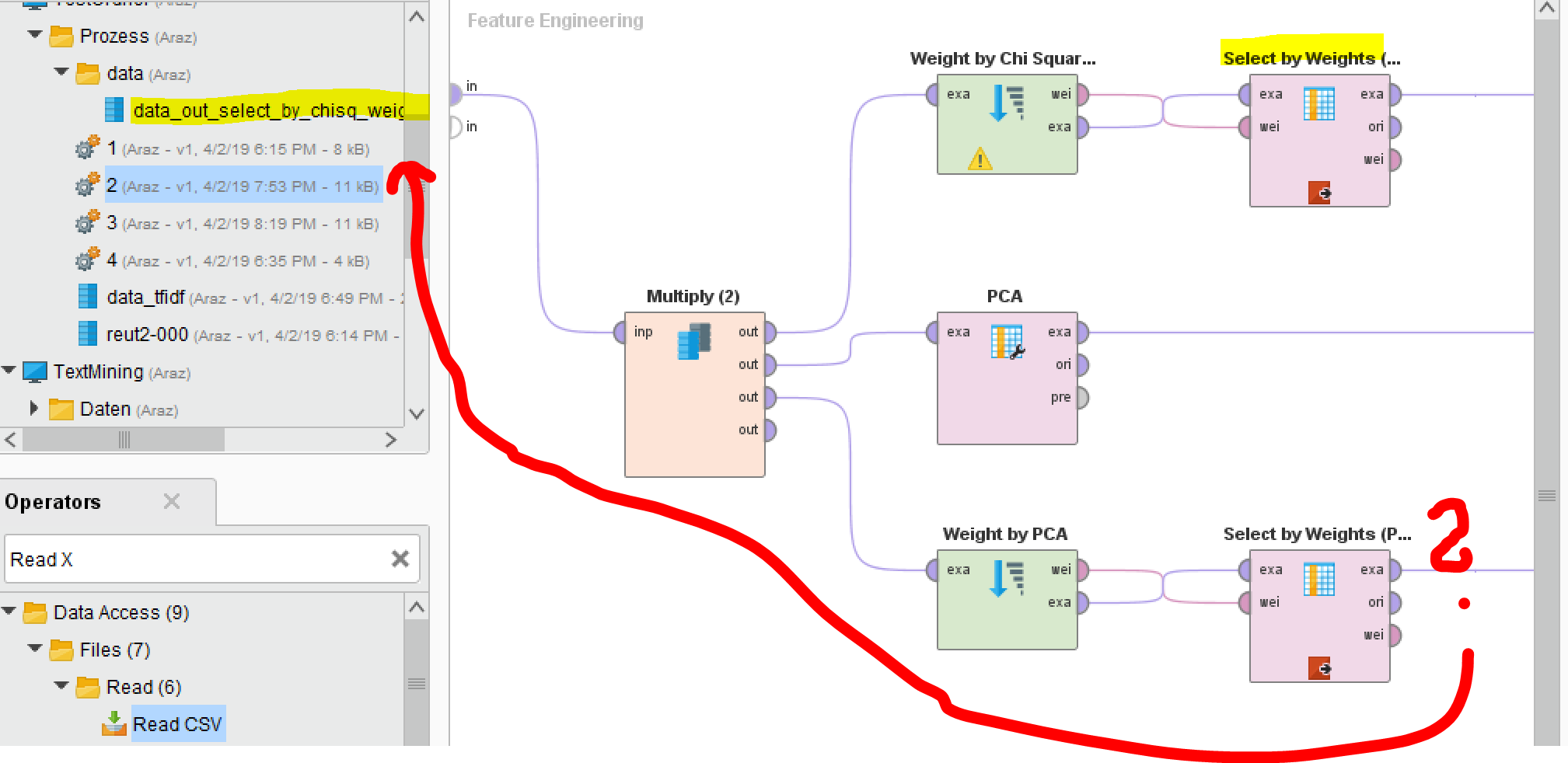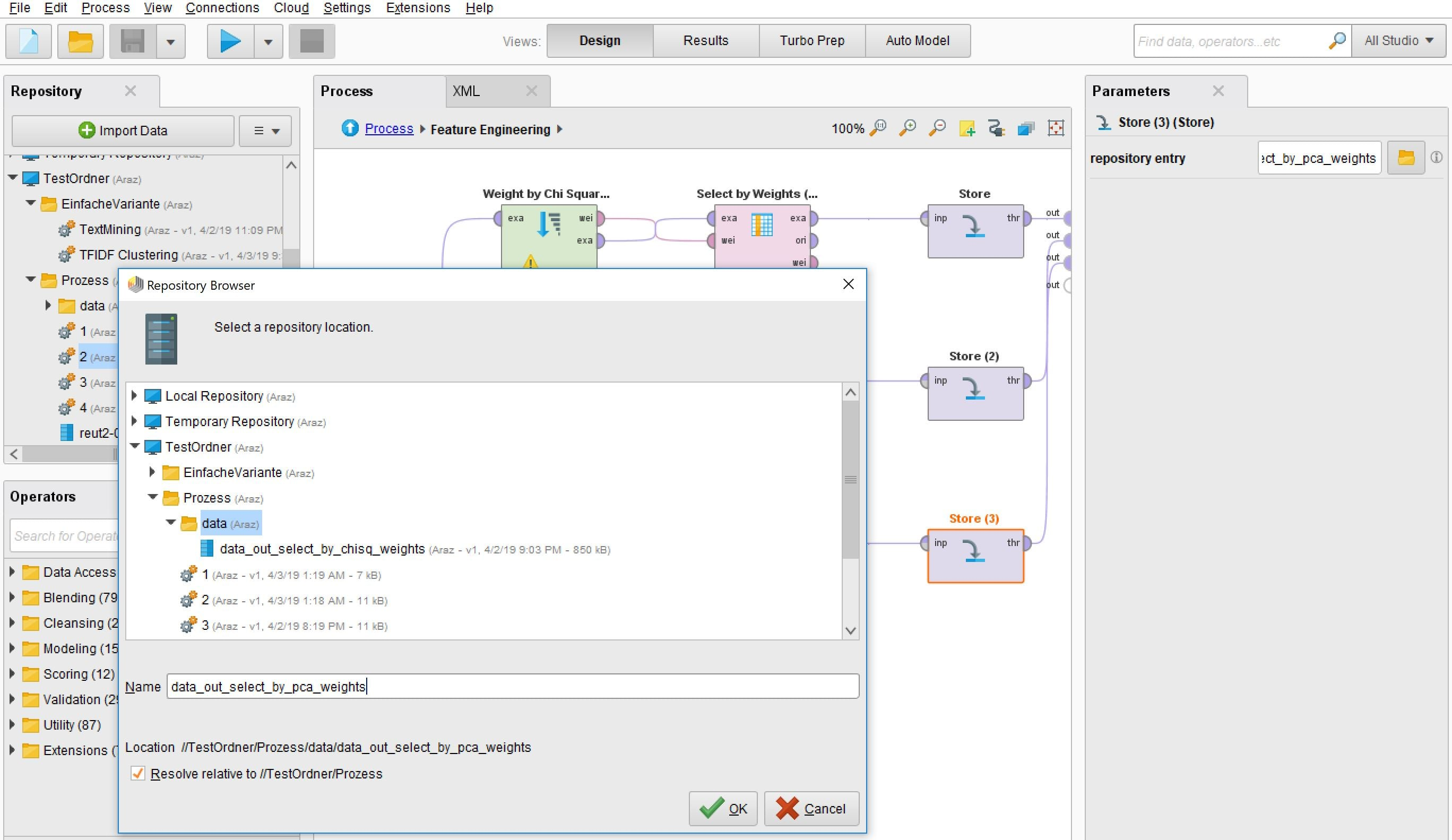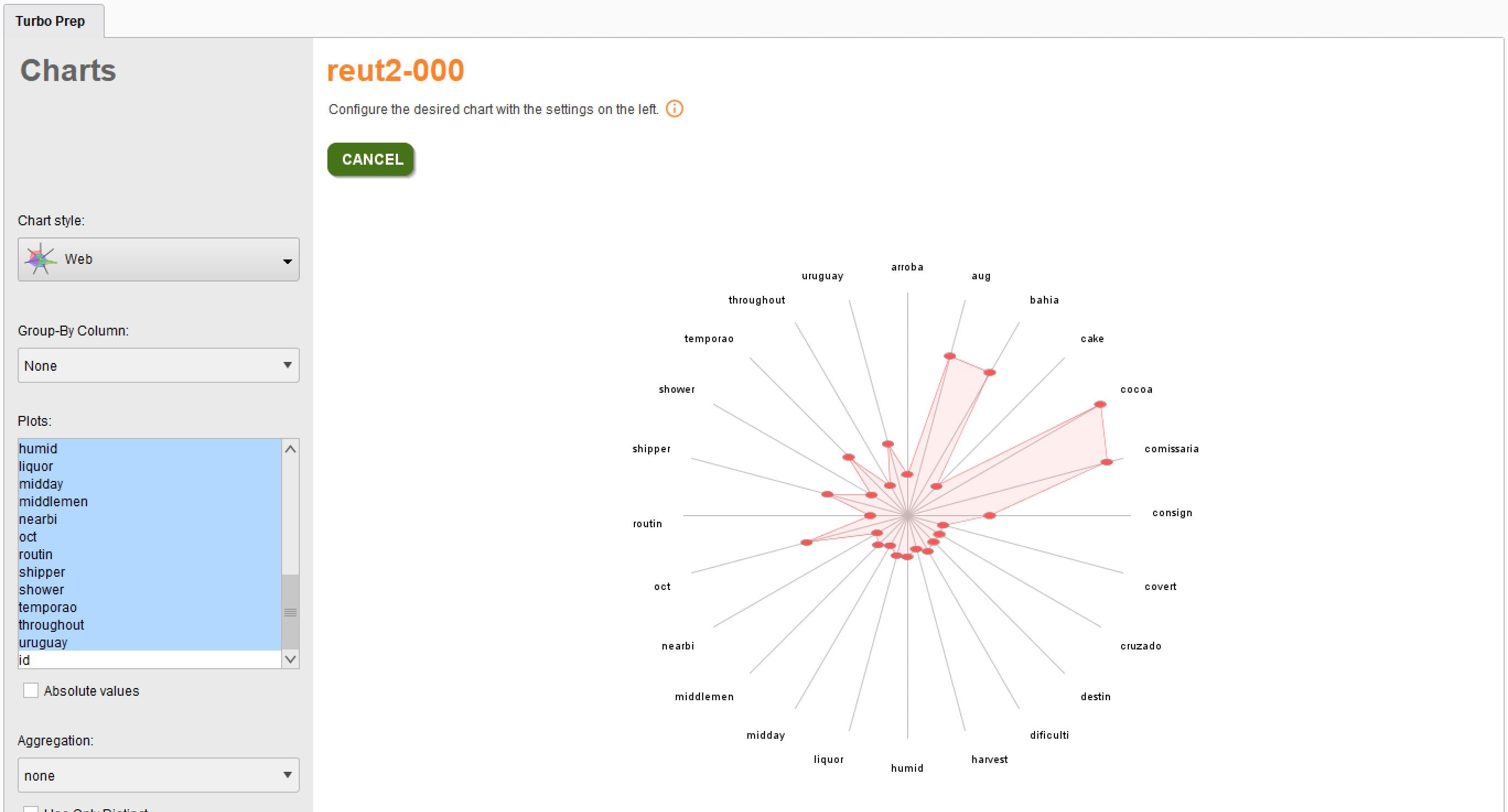# Data out selected by PCA

Member Posts: 33Contributor II
edited June 2019 in Help
```Hello,

I have configured the process here with the calculation via TF IDF. If I start the process, would the process actually give me the output as the Data_out_selected_by_pca or?
I visualized the whole thing again briefly (see screen)

BR,Flixport```(Screen)

• Member Posts: 33Contributor II
`No, I did not insert anything via drag & drop. Is that necessary? I ask because I did not insert anything at Chi Square. Of course, my input data is already ready as a CSV file, the attribute values ​​are also numeric, so I can not understand that unfortunatelyBR`

• Member Posts: 33Contributor II
the input is also correctly connected2.JPG 335K
• Member Posts: 33Contributor II
For sure @varunm1
i have inserted the folder for you to understand the relationships + the input csv data

BR

• Administrator, Employee, RapidMiner Certified Analyst, RapidMiner Certified Expert, Member Posts: 355RM Data Scientist
Hi @Flixport,

I suggest you add an operator for text vectorization. Otherwise the text data is not vectorized into TF-IDF vectors.

I used the Reuters data reut2-000, and added vectorization before feature selections. After text vecterization, we have almost 1000 attributes for the keywords, with weight by PCA and feature selection, we kept 50 attributes.

I have sample process for text classification using 20k+ Reuters news, PM me if you need.

Here is the process fixed for feature selection. Enjoy!
```<?xml version="1.0" encoding="UTF-8"?><process version="9.2.001">
<context>
<input/>
<output/>
<macros/>
</context>
<operator activated="true" class="process" compatibility="9.2.001" expanded="true" name="Process">
<parameter key="logverbosity" value="init"/>
<parameter key="random_seed" value="2001"/>
<parameter key="send_mail" value="never"/>
<parameter key="process_duration_for_mail" value="30"/>
<parameter key="encoding" value="UTF-8"/>
<process expanded="true">
<operator activated="true" class="retrieve" compatibility="9.2.001" expanded="true" height="68" name="Retrieve reut2-000" width="90" x="45" y="85">
<parameter key="repository_entry" value="//demo/TextMining/Reuters/reut2-000"/>
</operator>
<operator activated="true" class="select_attributes" compatibility="9.2.001" expanded="true" height="82" name="Select Attributes" width="90" x="179" y="85">
<parameter key="attribute_filter_type" value="subset"/>
<parameter key="attribute" value=""/>
<parameter key="attributes" value="|places|text"/>
<parameter key="use_except_expression" value="false"/>
<parameter key="value_type" value="attribute_value"/>
<parameter key="use_value_type_exception" value="false"/>
<parameter key="except_value_type" value="time"/>
<parameter key="block_type" value="attribute_block"/>
<parameter key="use_block_type_exception" value="false"/>
<parameter key="except_block_type" value="value_matrix_row_start"/>
<parameter key="invert_selection" value="false"/>
<parameter key="include_special_attributes" value="true"/>
</operator>
<operator activated="true" class="generate_id" compatibility="9.2.001" expanded="true" height="82" name="Generate ID" width="90" x="313" y="85">
<parameter key="create_nominal_ids" value="false"/>
<parameter key="offset" value="0"/>
</operator>
<operator activated="true" breakpoints="after" class="filter_examples" compatibility="9.2.001" expanded="true" height="103" name="Filter Examples" width="90" x="447" y="85">
<parameter key="parameter_expression" value=""/>
<parameter key="condition_class" value="custom_filters"/>
<parameter key="invert_filter" value="false"/>
<list key="filters_list">
<parameter key="filters_entry_key" value="places.does_not_equal.?"/>
</list>
<parameter key="filters_logic_and" value="true"/>
</operator>
<operator activated="true" class="set_role" compatibility="9.2.001" expanded="true" height="82" name="Set Role" width="90" x="581" y="85">
<parameter key="attribute_name" value="places"/>
<parameter key="target_role" value="label"/>
</operator>
<operator activated="true" class="model_simulator:text_vectorization" compatibility="9.2.001" expanded="true" height="103" name="Text Vectorization" width="90" x="715" y="85">
<parameter key="attribute_filter_type" value="single"/>
<parameter key="attribute" value="text"/>
<parameter key="attributes" value=""/>
<parameter key="use_except_expression" value="false"/>
<parameter key="value_type" value="nominal"/>
<parameter key="use_value_type_exception" value="false"/>
<parameter key="except_value_type" value="file_path"/>
<parameter key="block_type" value="single_value"/>
<parameter key="use_block_type_exception" value="false"/>
<parameter key="except_block_type" value="value_matrix_row_start"/>
<parameter key="invert_selection" value="false"/>
<parameter key="include_special_attributes" value="false"/>
<parameter key="keep original" value="false"/>
<parameter key="store training documents" value="true"/>
<parameter key="store scoring documents" value="false"/>
<parameter key="document class attribute" value=""/>
<parameter key="token split" value="\s+"/>
<parameter key="apply pruning" value="true"/>
<parameter key="max number of new columns" value="1000"/>
<description align="center" color="transparent" colored="false" width="126">convert text into tf-idf vectors</description>
</operator>
<operator activated="true" breakpoints="after" class="remove_correlated_attributes" compatibility="9.2.001" expanded="true" height="82" name="Remove Correlated Attributes" width="90" x="916" y="85">
<parameter key="correlation" value="0.8"/>
<parameter key="filter_relation" value="greater"/>
<parameter key="attribute_order" value="random"/>
<parameter key="use_absolute_correlation" value="true"/>
<parameter key="use_local_random_seed" value="false"/>
<parameter key="local_random_seed" value="1992"/>
</operator>
<operator activated="true" class="subprocess" compatibility="9.2.001" expanded="true" height="124" name="Feature Engineering" width="90" x="1117" y="85">
<process expanded="true">
<operator activated="true" class="multiply" compatibility="9.2.001" expanded="true" height="124" name="Multiply (2)" width="90" x="112" y="187"/>
<operator activated="true" class="weight_by_chi_squared_statistic" compatibility="9.2.001" expanded="true" height="82" name="Weight by Chi Squared Statistic" width="90" x="313" y="34">
<parameter key="normalize_weights" value="false"/>
<parameter key="sort_weights" value="true"/>
<parameter key="sort_direction" value="descending"/>
<parameter key="number_of_bins" value="10"/>
</operator>
<operator activated="true" breakpoints="after" class="select_by_weights" compatibility="9.2.001" expanded="true" height="103" name="Select by Weights (ChiSq)" width="90" x="514" y="34">
<parameter key="weight_relation" value="top k"/>
<parameter key="weight" value="10.0"/>
<parameter key="k" value="50"/>
<parameter key="p" value="0.1"/>
<parameter key="deselect_unknown" value="true"/>
<parameter key="use_absolute_weights" value="false"/>
</operator>
<operator activated="true" class="store" compatibility="9.2.001" expanded="true" height="68" name="Store" width="90" x="715" y="34">
<parameter key="repository_entry" value="//demo/TextMining/Reuters/tmp/DT cluster"/>
</operator>
<operator activated="true" class="principal_component_analysis" compatibility="9.2.001" expanded="true" height="103" name="PCA" width="90" x="313" y="187">
<parameter key="dimensionality_reduction" value="keep variance"/>
<parameter key="variance_threshold" value="0.8"/>
<parameter key="number_of_components" value="1"/>
</operator>
<operator activated="true" class="weight_by_pca" compatibility="9.2.001" expanded="true" height="82" name="Weight by PCA" width="90" x="313" y="340">
<parameter key="normalize_weights" value="false"/>
<parameter key="sort_weights" value="true"/>
<parameter key="sort_direction" value="ascending"/>
<parameter key="component_number" value="1"/>
</operator>
<operator activated="true" breakpoints="after" class="select_by_weights" compatibility="9.2.001" expanded="true" height="103" name="Select by Weights (PCA)" width="90" x="514" y="340">
<parameter key="weight_relation" value="top k"/>
<parameter key="weight" value="10.0"/>
<parameter key="k" value="50"/>
<parameter key="p" value="0.1"/>
<parameter key="deselect_unknown" value="true"/>
<parameter key="use_absolute_weights" value="true"/>
</operator>
<operator activated="true" class="store" compatibility="9.2.001" expanded="true" height="68" name="Store (3)" width="90" x="715" y="340">
<parameter key="repository_entry" value="//demo/TextMining/Reuters/tmp/data_out_select_by_pca_weights"/>
</operator>
<operator activated="true" class="store" compatibility="9.2.001" expanded="true" height="68" name="Store (2)" width="90" x="715" y="187">
<parameter key="repository_entry" value="//demo/TextMining/Reuters/tmp/data_out_pca"/>
</operator>
<connect from_port="in 1" to_op="Multiply (2)" to_port="input"/>
<connect from_op="Multiply (2)" from_port="output 1" to_op="Weight by Chi Squared Statistic" to_port="example set"/>
<connect from_op="Multiply (2)" from_port="output 2" to_op="PCA" to_port="example set input"/>
<connect from_op="Multiply (2)" from_port="output 3" to_op="Weight by PCA" to_port="example set"/>
<connect from_op="Weight by Chi Squared Statistic" from_port="weights" to_op="Select by Weights (ChiSq)" to_port="weights"/>
<connect from_op="Weight by Chi Squared Statistic" from_port="example set" to_op="Select by Weights (ChiSq)" to_port="example set input"/>
<connect from_op="Select by Weights (ChiSq)" from_port="example set output" to_op="Store" to_port="input"/>
<connect from_op="Store" from_port="through" to_port="out 1"/>
<connect from_op="PCA" from_port="example set output" to_op="Store (2)" to_port="input"/>
<connect from_op="Weight by PCA" from_port="weights" to_op="Select by Weights (PCA)" to_port="weights"/>
<connect from_op="Weight by PCA" from_port="example set" to_op="Select by Weights (PCA)" to_port="example set input"/>
<connect from_op="Select by Weights (PCA)" from_port="example set output" to_op="Store (3)" to_port="input"/>
<connect from_op="Store (3)" from_port="through" to_port="out 3"/>
<connect from_op="Store (2)" from_port="through" to_port="out 2"/>
<portSpacing port="source_in 1" spacing="0"/>
<portSpacing port="source_in 2" spacing="0"/>
<portSpacing port="sink_out 1" spacing="0"/>
<portSpacing port="sink_out 2" spacing="0"/>
<portSpacing port="sink_out 3" spacing="0"/>
<portSpacing port="sink_out 4" spacing="0"/>
</process>
</operator>
<connect from_op="Retrieve reut2-000" from_port="output" to_op="Select Attributes" to_port="example set input"/>
<connect from_op="Select Attributes" from_port="example set output" to_op="Generate ID" to_port="example set input"/>
<connect from_op="Generate ID" from_port="example set output" to_op="Filter Examples" to_port="example set input"/>
<connect from_op="Filter Examples" from_port="example set output" to_op="Set Role" to_port="example set input"/>
<connect from_op="Set Role" from_port="example set output" to_op="Text Vectorization" to_port="example set input"/>
<connect from_op="Text Vectorization" from_port="example set output" to_op="Remove Correlated Attributes" to_port="example set input"/>
<connect from_op="Remove Correlated Attributes" from_port="example set output" to_op="Feature Engineering" to_port="in 1"/>
<connect from_op="Feature Engineering" from_port="out 1" to_port="result 1"/>
<connect from_op="Feature Engineering" from_port="out 2" to_port="result 2"/>
<connect from_op="Feature Engineering" from_port="out 3" to_port="result 3"/>
<portSpacing port="source_input 1" spacing="0"/>
<portSpacing port="sink_result 1" spacing="0"/>
<portSpacing port="sink_result 2" spacing="0"/>
<portSpacing port="sink_result 3" spacing="0"/>
<portSpacing port="sink_result 4" spacing="0"/>
<description align="left" color="yellow" colored="false" height="278" resized="true" width="815" x="37" y="383">REDUKTION DER DIMENSIONALIT&amp;#196;T&lt;br/&gt;&lt;br/&gt;Hier geht hier darum, die Reduktion der Dimensionalit&amp;#228;t anzustreben. Zwei m&amp;#246;gliche Arten:&lt;br&gt;-- auf Basis PCA (braucht kein Zielvariable)&lt;br&gt;-- auf Basis ChiSquared (Zielvariable vorausus&lt;br&gt;Gibt es eine Zielvariable, so ist es m&amp;#246;glich nur diejenigen Felder zu behalten, die hohes Potenzial f&amp;#252;r ein Model haben.&lt;br&gt;&lt;br&gt;Schritte:&lt;br&gt;a. Input Daten TF-IDF&lt;br&gt;b. Non-TFIDF Felder rausfiltern: exchanges, org, people, usw.&lt;br&gt;c. Filter nur Datens&amp;#228;tze mit vollst&amp;#228;ndigen Werte &amp;#252;r Zielvariable&lt;br&gt;d. Entferne korrelierte TFIDF Felder&lt;br&gt;e. Verwende beiden Methoden zur Reduktion der Dimensionalit&amp;#228;t. Daten speichern.&lt;br&gt;&lt;br&gt;</description>
<description align="left" color="yellow" colored="false" height="58" resized="true" width="301" x="177" y="22">F&amp;#252;r die Reduktion der Dimensionalit&amp;#228;t bleibt eine Zielvariable und die TF-IDF Felder.</description>
</process>
</operator>
</process>
```

• Member Posts: 33Contributor II
The problem with this thing is that PCA can only work with integer and my input consists only of polynominal values. For the reduction of dimensionality I would like to use both methods. Is there perhaps a way out? Thank you

• 12Administrator, Moderator, Employee, RapidMiner Certified Analyst, Community Manager, Member, University Professor, PM Moderator Posts: 2,959Community Manager
@yyhuang can you pls send me that Reuters exampleset for the repo?• Member Posts: 33Contributor II
Hey all,

my process is ready. I can now extract the most important words of the Reuters News via an X of any ID. (See screen)
Does anyone want to look at my process, PN me.
I am looking forward to improvement potential.

BR(U can see here the most important words of a Message. Can I also add the title and date in the charts, is there a possibility?